Sie sind hier: ICP » R. Hilfer » Publikationen

# 5 Conservative Systems

[211.2.1] In classical mechanics the commutative algebra of observables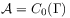is the algebra of continuous functions on phase space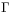, that vanish at infinitye (This is a footnote:) e This means that for each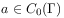and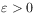there is a compact subset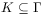such that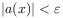for all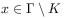.. [211.2.2] The characters (pure states) are point measures on phase space, and one has the isomorphism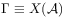. [211.2.3] By the Riesz representation theorem the states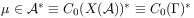in classical mechanics are probability measures on phase space. [211.2.4] Every state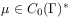gives rise to a probability measure space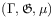where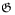is the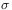-algebra of measurable subsets of phase space.

[211.3.1] Let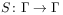be an invertible map such that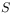and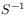are both measurable, i.e. such that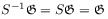where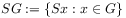for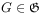. [211.3.2] The mapis called a measure preserving transformation and the measure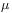onis called invariant under, if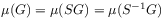for all. [page 212, §0]    [212.0.1] An invariant measure is called ergodic with respect to, if it cannot be decomposed into a convex combination of-invariant measures.

[212.1.1] Here and in the following the measure preserving transformation is the adjoint time evolution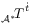which is denoted more briefly as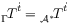. [212.1.2] Pure states (characters) are not invariant under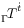. [212.1.3] Examples of invariant probability measures are furnished by the set of equilibrium states of a conservative system with Hamiltonian dynamics. [212.1.4] Ifis an equilibrium state of a conservative system then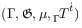is a measure preserving system.